# Difference between revisions of "Sinc function"

This article is about a particular function from a subset of the real numbers to the real numbers. Information about the function, including its domain, range, and key data relating to graphing, differentiation, and integration, is presented in the article.
View a complete list of particular functions on this wiki
For functions involving angles (trigonometric functions, inverse trigonometric functions, etc.) we follow the convention that all angles are measured in radians. Thus, for instance, the angle of$90\,^\circ$ is measured as$\pi/2$.

## Definition

This function, denoted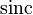$\operatorname{sinc}$, is defined as follows:$\operatorname{sinc} x := \left\lbrace \begin{array}{rl} 1, & x = 0 \\ \frac{\sin x}{x} & x \ne 0\end{array}\right.$

## Key data

Item Value
default domain all real numbers, i.e., all of$\R$.
range the closed interval$[\alpha,1]$ where$\alpha$ is approximately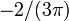$-2/(3\pi)$.
period none; the function is not periodic
horizontal asymptotes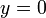$y = 0$, i.e., the$x$-axis. This is because$\lim_{x \to \pm \infty} \frac{\sin x}{x} = 0$, which in turn can be deduced from the fact that the numerator is bounded while the magnitude of the denominator approaches$\infty$.
local maximum values and points of attainment The local maxima occur at points$x$ satisfying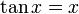$\tan x = x$ and$x in [2n\pi,(2n + 1)\pi]$ or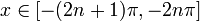$x \in [-(2n + 1)\pi,-2n\pi]$ for$n$ a positive integer.
There is an anomalous local maximum at$x = 0$ with value 1. Apart from that, the other local maxima occur at points of the form$\pm(2n\pi + \alpha_n)$ where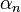$\alpha_n$ is fairly close to$\pi/2$ for all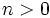$n > 0$. The local maximum value at this point is slightly more than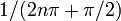$1/(2n\pi + \pi/2)$.
local minimum values and points of attainment The local minima occur at points$x$ satisfying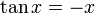$\tan x = -x$ and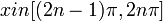$x in [(2n - 1)\pi,2n\pi]$ or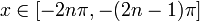$x \in [-2n\pi,-(2n - 1)\pi]$ for$n$ a positive integer.
The local minima occur at points of the form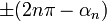$\pm(2n\pi - \alpha_n)$ where$\alpha_n$ is fairly close to$\pi/2$ for all$n > 0$. The local minimum value at this point is slightly less than$-1/(2n\pi - \pi/2)$.
points of inflection Fill this in later
important symmetries The function is an even function, i.e., the graph has symmetry about the$y$-axis.
derivative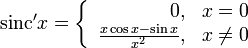$\operatorname{sinc}'x = \left\lbrace \begin{array}{rl} 0, & x = 0 \\ \frac{x \cos x - \sin x}{x^2}, & x \ne 0\\\end{array}\right.$
antiderivative the sine integral (this is defined as the antiderivative of the sinc function that takes the value 0 at 0)
power series and Taylor series The power series about 0 (which is also the Taylor series) is$\sum_{k=0}^\infty \frac{(-1)^kx^{2k}}{(2k + 1)!} = 1 - \frac{x^2}{3!} + \frac{x^4}{5!} - \dots$
The power series converges globally to the function.

## Graph

Below is a graph of the function for the domain restricted to$[-3\pi,3\pi]$:

The picture is a little unclear, so we consider an alternative depiction of the graph where the$x$-axis and$y$-axis are scaled differently to make it clearer:

## Differentation

### First derivative

Computation at$x = 0$: Here, we need to compute the derivative using first principles, as a limit of a difference quotient:$\operatorname{sinc}' 0 := \lim_{x \to 0} \frac{\operatorname{sinc} x - \operatorname{sinc} 0}{x - 0} = \lim_{x \to 0} \frac{(\sin x)/x - 1}{x} = \lim_{x \to 0} \frac{\sin x - x}{x^2}$

This limit can be computed in many ways. For instance, it can be computed using the L'Hopital rule: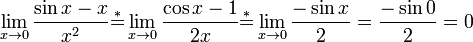$\lim_{x \to 0} \frac{\sin x - x}{x^2} \stackrel{*}{=} \lim_{x \to 0} \frac{\cos x - 1}{2x} \stackrel{*}{=} \lim_{x \to 0} \frac{-\sin x}{2} = \frac{-\sin 0}{2} = 0$

WARNING ON ERRONEOUS DIFFERENTIATION APPROACH: Suppose a function is given a separate definition at an isolated point from the definition at points surrounding it (on its immediate left or immediate right). To compute the derivative, it is not correct to simply differentiate the definition at the point and arrive at the derivative. Rather, we need to compute the derivative from first principles as a limit of a difference quotient, where the function value at the point is taken as the specified value and the function value at nearby points is given by the expression used to define the function around the point.

Computation at$x \ne 0$: At any such point, we know that the function looks like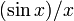$(\sin x)/x$ in an open interval about the point, so we can use the quotient rule for differentiation, which states that: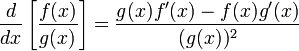$\frac{d}{dx}\left[\frac{f(x)}{g(x)}\right] = \frac{g(x)f'(x) - f(x)g'(x)}{(g(x))^2}$

In this case,$f(x) = \sin x$ and$g(x) = x$, so we get:$\frac{d}{dx}(\operatorname{sinc}\ x) = \frac{x \cos x - \sin x}{x^2}$